# Surface Area and VolumePage 1

#### WATCH ALL SLIDES

Slide 1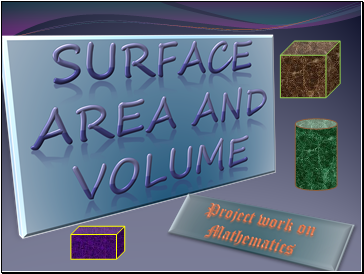## Surface area and volume

Project work on Mathematics

Slide 2CUBE :- A solid three-dimensional geometric figure bounded by six planes; each of the six sides or faces of a cube is a

a1

a2

a3

Slide 3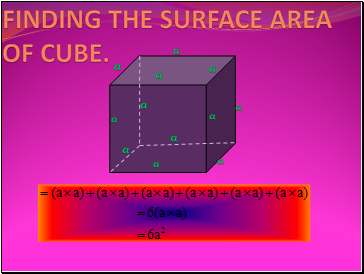Finding the surface area of cube.

a

a

a

a

a

a

a

a

a

a

a

a

Slide 4FINDING LATERAL SURFACE AREA OF CUBE:-

Slide 5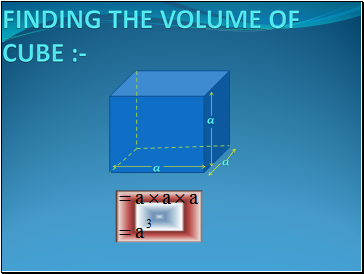Finding the volume of cube

a

a

a

Slide 6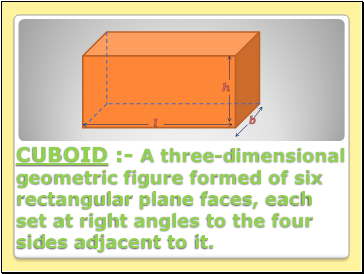CUBOID :- A three-dimensional geometric figure formed of six rectangular plane faces, each set at right angles to the four sides adjacent to it.

b

h

l

Slide 7Finding total surface area of cuboid

b

h

l

l

l

l

h

h

h

b

b

b

Slide 8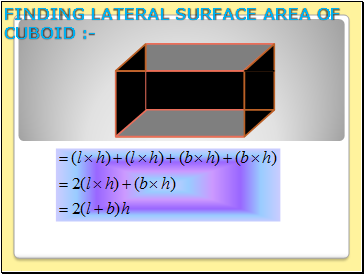FINDING LATERAL SURFACE AREA OF CUBOID :-

Slide 9FINDING VOLUME OF CUBOID:-

b

h

l

Slide 10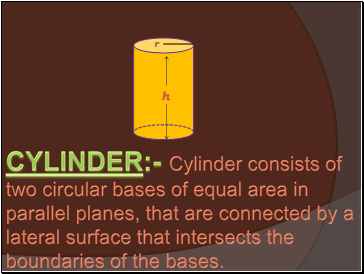CYLINDER:- Cylinder consists of two circular bases of equal area in parallel planes, that are connected by a lateral surface that intersects the boundaries of the bases.

h

r

Slide 11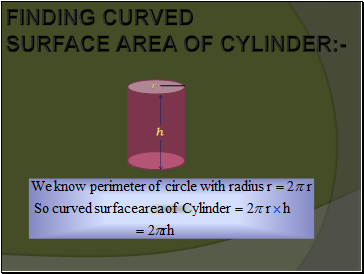FINDING CURVED

SURFACE AREA OF CYLINDER:-

h

r

Slide 12TOTAL SURFACE AREA OF CUBOID :-

h

r

Slide 13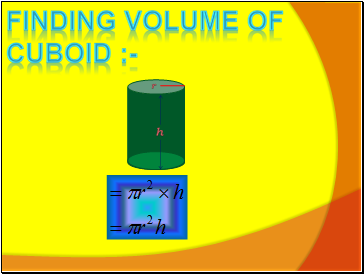Finding Volume of cuboid :-

h

r

Slide 14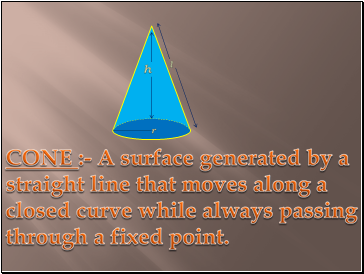CONE :- A surface generated by a straight line that moves along a closed curve while always passing through a fixed point.

h

r

l

Slide 15h

r

l

FINDING CURVED SURFACE AREA OF

CONE :-

Slide 16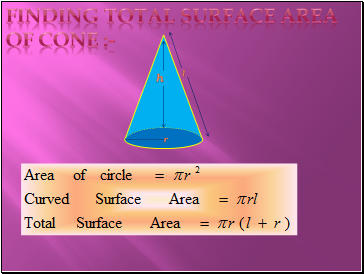h

r

l

Finding total surface area of cone :-

Go to page:
1  2